### Home > MC2 > Chapter 6 > Lesson 6.2.2 > Problem6-89

6-89.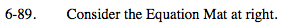Refer to the Math Notes from Lesson 6.2.1 for help.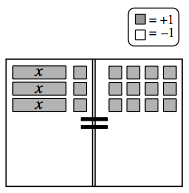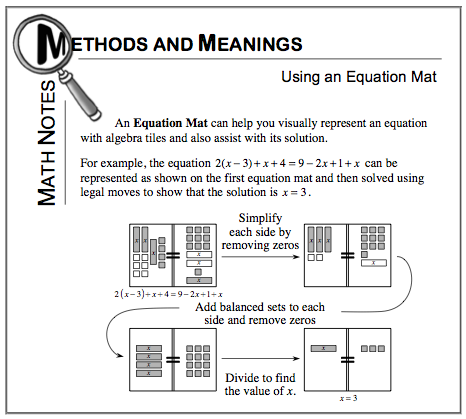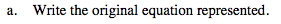Count the number of x-tiles and unit tiles on each sideof the equation, then turn that into an equation.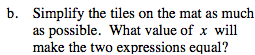Remove matching sets of tiles from each side.

Now you are left with 3 x-tiles on the left and 9 unit tileson the right. Divide each side by 3 to find the value of x.

x = 3